# Bohr model of Potassium atom - How to draw Potassium(K) Bohr-Rutherford diagram?

Home  > Chemistry Article > Potassium Bohr model

The Bohr Model of Potassium(K) has a nucleus that contains 20 neutrons and 19 protons. This nucleus is surrounded by four-electron shells named K-shell, L-shell, M-shell, and N-shell. The outermost shell in the Bohr diagram of Potassium contains only 1 electron that also called valence electron.

 Name Potassium Bohr Model Number of neutrons 20 Number of protons 19 Number of electrons 19 Total electron shells 4 Electron in the First shell(K) 2 Electrons in the Second shell(L) 8 Electrons in the Third shell(M) 8 Electrons in the Fourth shell(N) 1 Total valence electrons in Potassium 1
Page Contents

## How to draw Bohr Model of Potassium(K)?

Bohr model describes the visual representation of orbiting electrons around the small nucleus. It used different electron shells such as K, L, M, N…so on. These shells hold a specific number of electrons, the electron shell which is closest to the nucleus has less energy and the electron shell which is farthest from the nucleus has more energy.

Bohr diagram is very interesting and easy to draw. Here, we will draw the Bohr diagram of the Potassium atom with some simple steps.

## Steps to draw the Bohr Model of Potassium atom

1. Find the number of protons, electrons, and neutrons in the Potassium

Protons are the positively charged particles and neutrons are the uncharged particles, both these are constituents of the atom nuclei. Electrons are the negatively charged particles that orbit the nucleus of an atom

To find the number of protons an atom contains, just look at their atomic number.

If the atomic number of atom is 41, then proton will also be 41, if atomic number is 43, then proton will also be 43.

So, the atomic number for Potassium is 19, hence, the number of protons in the Potassium atom is also 19.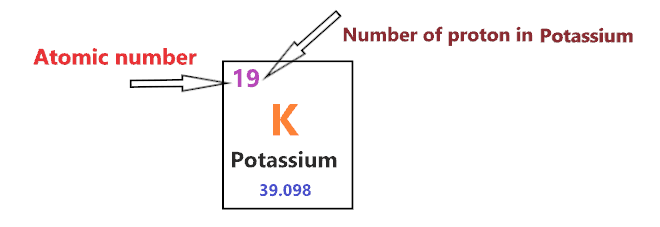Now, to determine the number of neutrons in an atom, use this formula.

⇒ Number of neutrons in atom = Atomic mass of the atom(rounded to the nearest whole number) – Number of proton in an atom

For example, An atom have 27.076 atomic mass and 7 protons.

Then, to find the number of neutron, round the atomic mass to the near whole number, so, atomic mass 27.076 round to 27.

= (27 – 7 protons) = 20 number of neutrons in the atom

Now, To get the number of neutrons in a Potassium atom, look at its atomic mass which is 39.098 rounded to 39, and the number of protons in Potassium is 19.

∴ Hence, the number of neutrons in Potassium atom = (39 – 19) = 20It should be noted that “The number of electrons in a neutral atom is equal to the number of protons”.

So, the Potassium atom is neutral, hence, its number of electrons will be equal to the number of protons which is 19 as we already discussed.

⇒ The number of electrons in Potassium atom = 19

⇒ The number of protons in Potassiumatom = 19

⇒ The number of neutrons in a Potassium atom = 20

2. Draw the nucleus of an atom

A nucleus is a dense and small region that contains the number of protons and neutrons of an atom.

In this step, we have to draw a small circle that consists of a number of protons and the number of neutrons of a Potassium atom.3. Draw the First electron shell

“An electron shell may be thought of as an orbit followed by electrons around an atom’s nucleus.”

The first electron shell is also called the K-shell, this is the closest shell to the nucleus of an atom and can hold a maximum of two electrons.

As we know, the Potassium atom has a total of 19 electrons. So, put two electrons from it, in the first shell, next to each other.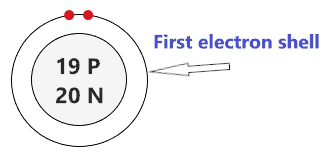We have successfully drawn the first shell of the Potassium atom that can hold 2 electrons. As a Potassium atom has a total of 19 electrons, and from 19 electrons we have used two electrons in the first shell.

∴ (19 – 2) = 17 electrons

Therefore, we are left with 17 electrons, let’s put them in the next shells of the Potassium atom.

4. Draw the Second electron shell

The second shell also called the L-shell that can hold a maximum of 8 electrons. This shell is drawn after the first electron shell.

In the second electron shell, the electrons are added one at a time, starting from the top position then going in a clockwise direction.

In second shell, electrons are added one at a time in clockwise direction as a clock position – 12 o’clock, 3 o’clock, 6 o’clock, 9 o’clock positions.Once you place the electrons one at a time to each of the four sides(Top – Right – Bottom – Left], start pairing or doubling them.

So, we have 17 remaining electrons of a Potassium atom, and the 2nd shell can only hold a maximum of 8 electrons.

Therefore, put the 8 electrons of the Potassium atom in the 2nd electron shell, start from the top position, put electrons one at a time, and go in a clockwise direction(Top – Right – Bottom – Left). And, finally, pair them up.As we have a total of 19 electrons for the Potassium atom and we placed 2 electrons in the first shell and 8 electrons in the second shell, hence, we are left with 9 electrons more.

Let’s place it in the next shells.

5. Draw the Third electron shell

The third shell is also called the M-shell and it has the capacity to hold up to 18 electrons but, for the elements in the first few periods, the third shell only holds up to 8 electrons.

“The third shell can be considered to hold 8 or 18 electrons but in total the third shell can hold 18 electrons.”

For the first few elements, say, till atomic number 20 on the periodic table, the third shell holds up to 8 electrons.

⇒ For atomic number 20 or less than 20, the third shell can hold up to maximum of 8 electrons.

⇒ For the atomic number more than 20, say, atomic numbers 21, 22, 23, 24, 25, 26, 27,…….etc., the third shell is filled with more than 8 electrons and up to maximum of 18 electrons.

For the Potassium atom, the third shell is filled with the same procedure as the second shell and it will hold up to 8 electrons as its atomic number is 19.

So, we have left with 9 electrons of a Potassium atom and the third shell will hold up to a maximum of 8 electrons.

Therefore, put the 8 electrons of the Potassium atom in the 3rd electron shell, start from the top position, put electrons one at a time, and go in a clockwise direction(Top – Right – Bottom – Left). And, finally, pair them up.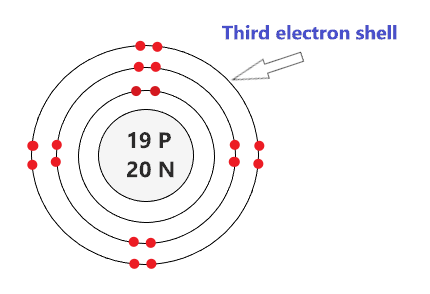Now we are just left with only 1 electron more, let’s put it in the fourth shell.

6. Draw the Fourth electron shell

Here, draw the fourth electron shell and put the one remaining electron of Potassium at the top position.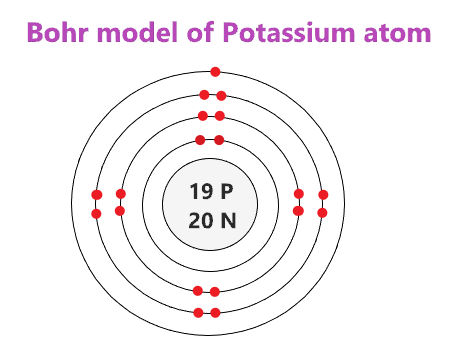### Bohr model of Potassium atom

That’s all, this is our Bohr model of the Potassium atom that contains 19 protons and 20 neutrons in the nucleus region, and 19 electrons are orbited around the nucleus, two electrons in the first shell, eight electrons in the second shell, eight electrons in the third shell, and one electron in the fourth shell.

## Find Valence electron of Potassium through its Bohr diagram

Valence electrons are found in the outermost shell of an atom and they can take participate in the formation of a chemical bond. These electrons have more energy compare to the inner shell electrons.

From the Bohr diagram of an atom, we can easily find the number of valence electrons in an atom by looking at its outermost shell.

So, we have to find a valence electron in the Potassium atom, for this, look at its Bohr diagram.

Bohr’s diagram of Potassium has four electron shells (K, L, M, N), the inner shell is K-shell and the outermost shell is N-shell.

Hence, the electrons found in the N-shell of the Potassium atom are its valence electrons because it is the outermost shell that also called the valence shell.

The N-shell or outer shell of the Potassium Bohr model contains only 1 electron, therefore, the number of the valence electrons in the Potassium atom is also 1.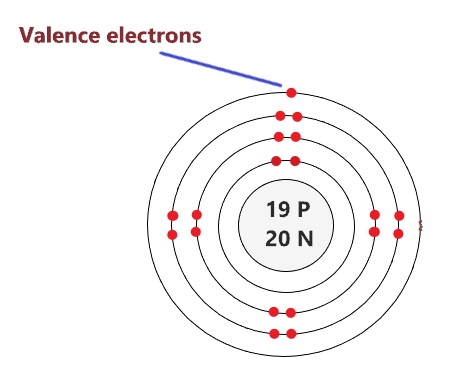## Electron dot diagram of a Potassium atom

Electron dot diagram also called lewis structure which represents the valence electrons of atoms.

As, from the Bohr diagram of Potassium, we got to know, it has only 1 valence electron.

So, just represent the 1 valence electron around the Potassium atom as a dot.## The electron configuration of Potassium

Potassium has an atomic number of 19 and it contains a total number of 19 electrons. From the Bohr model of Potassium, we know, 2 electrons are in the K-shell, 8 electrons are in the L-shell, 8 electrons are in the M-shell and 1 electron is in the N-shell.

So, based on the shell, the electronic configuration of the Potassium atom is [2, 8, 8, 1].

Or the electronic configuration of Potassium is 1s22s22p63s23p64s1 since it contains a total of 19 electrons.

## FAQ

### How many electron shells a Potassium Bohr model contains?

Electron shell also called energy level, you can find the number of electron shells for an element by knowing its period number in the periodic table.

The elements or atoms in the first period of the periodic table have one energy level or one electron shell, same as, the elements in the second period have two energy levels or two-electron shells and so on…

So, the Potassium atom belongs to the 4th Period in the periodic table, hence, the number of electron shells for the Bohr model of Potassium will be 4 (K-shell, L-shell, M-shell, and N-shell).

### What is the outer shell of the Bohr diagram of the Potassium atom?

The outermost shell also called the valence shell, which contains valence electrons of the atom.

According to the Bohr diagram of Potassium, the outer shell is N-shell which contains only one valence electron.

## Properties of Potassium

1. It appears as silvery-grey metal.
2. It has a boiling point of 759 °C and a melting point of 63.5 °C.
3. Its oxidation state varies from -1 to +1.
4. Its electronegativity is 0.82 at the Pauling scale.
5. It occurs in many minerals such as orthoclase.

## Summary

• The Bohr model of Potassium(K) is drawn with four electron shells, the first shell contains 2 electrons, the second shell contains 8 electrons, the third shell contains 8 electrons and the fourth shell contains 1 electron.
• Potassium is neutral and its atomic number is 19, hence, the number of protons and electrons available for its Bohr diagram is also 19.
• The number of neutrons for the Bohr diagram of Potassium can be found by subtracting the number of protons from the atomic mass(rounded to the nearest whole).
• The electron configuration of Potassium in terms of the shell is [2, 8, 8, 1], or in normal form, it is [Ar] 4s¹.
##### Subscribe to Blog via Email

Join 2 other subscribers

Share it...